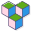CppBuzz# Interview Questions on Operators in C++ : (19)

Question: 1

(A) Scope Resolution Operator
(B) Conditional Operator
(C) Ternary Operator
(D) if else o/p

Compiler
Level: Beginner Category: operators
[Posted by: | ]

Question: 2

(A) 2 Bytes
(B) 4 Bytes
(C) 1 Byte
(D) Depends on Compiler

Compiler
Level: Beginner Category: operators
[Posted by: | ]

Question: 3

(A) +
(B) -
(C) *
(D) ::

Compiler
Level: Beginner Category: operators
[Posted by: | ]

Question: 4

(A) +
(B) -
(C) ++
(D) *

Compiler
Level: Beginner Category: operators
[Posted by: | ]

Question: 5

(A) 11
(B) 10
(C) Error
(D) 0

Compiler
Level: Beginner Category: operators
[Posted by: | ]

Question: 6

(A) 10
(B) 11
(C) Compilation Error

Compiler
Level: Beginner Category: operators
[Posted by: | ]

Question: 7

(B) write()
(C) both A and B

Compiler
Level: Practitioner Category: operators
[Posted by: | ]

Question: 8

(A) *
(B) /
(C) %
(D) all have same precedence

Compiler
Level: Practitioner Category: operators
[Posted by: | ]

Question: 9

(A) ()
(B) =
(C) *
(D) ++

Compiler
Level: Beginner Category: operators
[Posted by: | ]

Question: 10

(A) 4.5
(B) 4.0
(C) 4
(D) Compilation Error

Compiler
Level: Beginner Category: operators
[Posted by: | ]

Question: 11

(A) 10
(B) 11
(C) 12
(D) Not defined

Compiler
Level: Beginner Category: operators
[Posted by: | ]

Question: 12

(A) 111011
(B) 111111
(C) 101011
(D) 101010

Compiler
Level: Beginner Category: operators
[Posted by: | ]

Question: 13

(A) *
(B) ++
(C) +
(D) -

Compiler
Level: Beginner Category: operators
[Posted by: | ]

Question: 14

(A) c1=-7,c2=-4
(B) c1=-7,c2=-3
(C) c1=-4,c1=b-3
(D) c1=-6,c2=-6

Compiler
Level: Practitioner Category: operators
[Posted by: | ]

Question: 15

(A) <
(B) <<
(C) ->
(D) >>

Compiler
Level: Beginner Category: operators
[Posted by: | ]

Question: 16

(A) True
(B) False

Compiler
Level: Practitioner Category: operators
[Posted by: | ]

Question: 17

(A) re-assigned
(B) reformatted
(C) constructed

Compiler
Level: Practitioner Category: operators
[Posted by: | ]

Question: 18

(A) True
(B) False

Compiler
Level: Practitioner Category: operators
[Posted by: | ]

Question: 19

(A) True
(B) False

Compiler
Level: Practitioner Category: operators
[Posted by: | ]